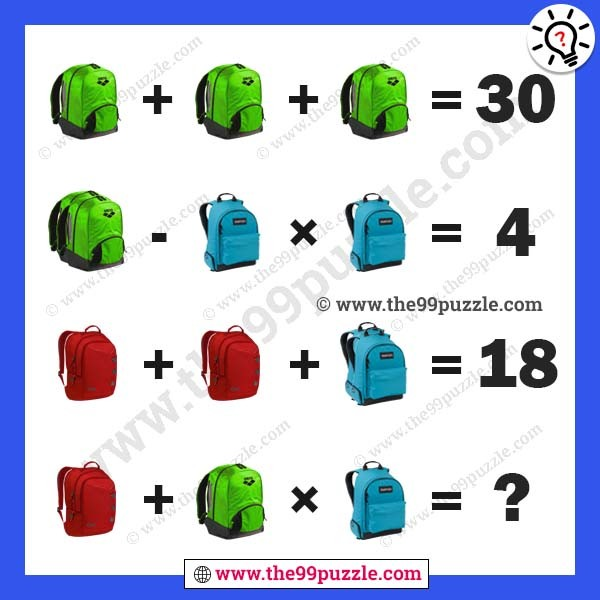# Bag pictures puzzles math equation for genius – Puzz187

Bag pictures puzzles math equation for genius. This math picture puzzle is viral on many social media. Most of the people failed to answer these viral puzzles. If you are a genius minded you can find out the value of viral math picture puzzles.###### Explanation:

Green Bag+Green Bag+Green Bag=30

(Green Bag+Green Bag)-Blue Bag×Blue Bag=4

Red Bag+Red Bag+(Blue Bag+Blue Bag)=18

Red Bag+Green Bag×Blue Bag= ?

10+10+10 = 30

(10+10)-4×4 = 4

5+5+(4+4) = 18

5+10×4 = 45